Pathways To Learning... Since 2005 Hong Kong Registered School 566985

# S - saddle point to system of equations - Mathematics Dictionary

A   B   C   D   E   F   G   H   I   J   K   L   M   N   O   P   Q   R   S   T   U   V   W   X   Y   Z

saddle point: A non-extremal turning point.

salient angle: An interior angle os a geometric figure that is locally convex. An interior angle less than 180°.

salient point: A self-intersecting point on a curve with more than one tangent at that contact point.

sample: A subset of the population used to produce statistics in order to infer results on the population.

sample correlation coefficient: Correlation coefficient on a sample as an estimator of the population correlation coefficient.

sample space: The set of all possible outcomes from an experiment.

sample statistic: Another class="d-title" name for a statistic.

sampling distribution: The probability distribution of a statistic on a random sample from a population.

sampling error: The difference between an estimator (sample statistic) and the corresponding population parameter.

scalar: 1. Having the properties of a scalar quantity.

2. A short form of the term scalar quantity.

scalar matrix: A matrix which is a scalar multiple of an identity matrix.

scalar product: Also called the dot product, due to the way it is denoted to distinguish from other types of multiplication of vectors. It is binary operation on vectors whose value is a scalar quantity.

scalar quantity: A quantity with only magnitude and not direction.

scale factor: The ratio of sides of the image of an enlargement, to the pre-image.

scalene triangle: A triangle where no two sides are of equal length.

scatter diagram: The representation of a data set of 2 variables by points (one for each data point) where the x, y coordinates represent the values in the 2 variables for the data point.

scientific notation: Exponential notation in base 10, used to represent very large or very small values, or simply to provide a standard for easy comparison with very large and/or very small values.

s.d.: A shorthand for standard deviation .

s.e.: A shorthand for standard error.

sec: A shorthand for the trigonometric function of secant.

secant: 1. A line that cuts a curve without being tangential at 2 points of the curve or more.

2. A trigonometric function defined to be the reciprocal of the trigonometric function of cosine.

secant method: An iterative process for finding the numerical approximation of the root of an equation by forming a secant with 2 estimates x0 and x1 of the root, then (repeatedly) considering the x-intercept of the secant to be one of the new points, together the higher indexed of the older 2 points forming a new secant.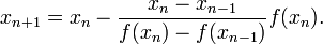sech: The hyperbolic function of hyperbolic secant.

second: 1. A unit for the measure of angle that is one sixtieth (1/60) of a minute, or one three-thousand-six-hundredth (1/3600) of a degree.

2. A unit of measure of time that is one sixtieth (1/60) of a minute, or one three-thousand-six-hundredth (1/3600) of an hour.

second derivative: The derivative of the derivative of a function f(x), denoted f''(x). Since the derivative (also known as a derived function) of a function is itself a function, therefore the derivative may well have it own derivative - this is known as the second (order) derivative.

second derivative test: A method of classifying a turning point (as a maximum, minimum or inflection) of a function, by the value of the second derivative at the turning point. Note that the second derivative test alone does not definitively classify a stationary point as an inflection. The test only definitively classify a stationary point as a maximum, minimum, or indeterminate (for the test), additional criteria is needed for the classification of an inflection.

secondary diagonal: The elements on the diagonal (that is not the leading diagonal) of a matrix, also known as the anti-diagonal. For an nxn matrix, the elements mi,j where i+j=n+1.

secondary parts: The median, altitude, orthocenter etc., i.e. any derived parts of a triangle which is not one of the 3 sides or one of the 3 interior angles.

second order differential equation: A differential equation with second derivatives being derivatives of the highest order which appears in the equation.

section: The plane figure that is the intersection of a plane and a solid. Also known as a plane section.

sector: The part of a circle between the circumference and two radii. Any pair of radii (which do not form a diameter together) dividing a circle into 2 parts has the larger part called the major sector and the smaller part the minor sector.

segment: The part of a circle between the circumference and a chord of the circle. Any chords (that is not a diameter) dividing a circle into 2 parts has the larger part called the major segment and the smaller part the minor segment.

self similar: Having the property of self-similarity.

self-similarity: A property of a geometric figure where the figure (or part of it) resembles itself (or another part of it) under elementary transformations, such as rotation, reflection, translation, enlargement etc.

semicircle: Half a circle formed by half of the circumference with the diameter to form a shape whose perimeter is a closed curve.

semi-interquartile range: The value of half of the inter-quartile range.

semimajor axis: 1. A radius of the smallest circle circumscribing a given ellipse where one of the endpoint is on the ellipse.

2. The radius of the smallest sphere circumscribing a given ellipsoid where one of the endpoints is on the ellipsoid.

3. A line segment between the centre and a vertex of the hyerbola.

semimean axis: The line segment between the centre of an ellipsoid and one of the intersections of the ellipsoid and a line perpendicular to both the semimajor and semiminor axis through the centre.

semiminor axis: 1. The radius of the largest circle incribed in a given ellipse where one of the endpoints is on the ellipse.

2. The radius of the largest sphere incribed in a given ellipsoid where one of the endpoints is on the ellipsoid.

3. A line segment between the centre of the hyperbola and a point on the line through the centre perpendicular to the semimajor axis, where a line through this point parallel to the semimajor axis intersect with both asymptotes at points such that these points on the asymptotes are on the tangent of the vertices.

sense: 1. The "direction" of an inequality. i.e. whether an inequality is one where the former is larger than the latter (the latter less than the former) or the former less than the latter (the latter larger than the former).

2. The direction of clockwise and anticlockwise from some reference axis/axes.

Separation: The partition of a set into 2 subsets. i.e. The formation fo 2 new sets, both subsets of the specified set, such that their intersection is the empty set and their union is the specified set.

sequence: A set of terms (which may be numbers, algebraic expressions or any mathematical objects, usually of the same type) with an order attached to the members. i.e. a list of mathematical objects

series: The summation of all the terms in a sequence a1, a2, a3, a4, ....

where the Nth partial sum can be calculated byand a series is considered convergent if the sequence of the partial sums of the series convergent.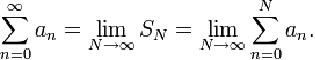set: The collection of objects considered as a whole. The collection is called a set and the objects within a collection members or elements.

In general, there are no orders attached to members of a set, and the same object cannot appear more than once within a set (repeated objects are simply ignored).

A set-like mathematical object which has an order attached to the collection of elements is called an ordered n-tuple, n-tuple or list.

A set-like mathematical object which allows identical membership is called a multiset.

set braces: Braces (the symbols { and }, always used together, in that order) used to indicate exhaustively (if completely defined) the elements of a set. e.g. {a, b} denotes a set of exactly 2 elements, X = {a, b, c, e} implies that d is not a member of X, and { } is the empty set (the set with no members).

set theory: The mathematical study of collection of objects (called members or elements) called sets.

sexagesimal: Of or related to the number 60. e.g. sexagesimal numbers - numbers in base 60.

sextic: Of degree six or sixth order.

shear: A linear transformation where points are translated parallel to the line of invariant points, by an amount proportional to the distance between the point and the line, where additionally, points on the same sides of the line are translated in the same direction and points on opposite sides of the line are translated in opposite directions.

shell method: A method for evaluating the volume of a solid of revolution, by integrating with respect to a variable whose axis is perpendicular to the axis of rotation. (As opposed to the disk method, where the axis of the variable with respect to which the function is integrated, is parallel to the axis of rotation.).

short arc: Also known as the minor arc of a circle.

short radius: The radius of the largest circle inscribed in a polygon.

SI: International System of Units.

side: 1. An edge of a (mostly 2-dimensional) geometric figure.

2. A face of a 3-dimensional geometric figure.

sieve of Eratosthenes: A prime number sieve, it is an algorithm for finding prime numbers.

First list all positive integers above 1 and circle the first number (which is 2) and cross out all subsequent mulitples of that number (i.e. all even numbers). Then repeat the steps of circling the first number which is not crossed out and cross out all multiples of this newly circled number (although there is no need to cross out any numbers already crossed out). By repeating these steps, the circled numbers are all prime numbers and all prime numbers will be found this way, provided that the list is long enough, and that the steps have been repeated enough number of times.

sigma function: 1. The Weierstrass sigma-function.

2. The divisor function, which is defined to be the sum of the positive factors of a specified number. e.g. σ(24) = 1+2+3+4+6+8+12+24=60.

sigma notation: A summation through the use of the summation sign.

sigmoid curve: A type of curves whose shape captures some or all of a few properties such as strictly monotone, real differentiable, defined over the real line, asymptotic end behaviours (at both end) and having a first derivative in the shape of a single bump.

A prime example of a sigmoidal curve is the graph of the logistic function, as seen below.

.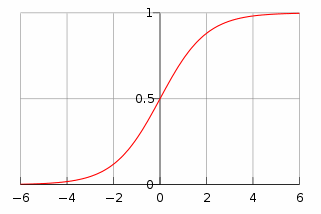signed minor: Also known as cofactors. It is the minor of an element of a matrix which is augmented by + or - signs in a chequered pattern. Specifically an element of the matrix of cofactors ci,j=(-1)i+jmi,j where mi,j is an element of the matrix of minors.

signed number: Also known as a directed number.

significance level: The probability below which an observation as extreme (or more) would have to be, by first assuming the null hypothesis, before the assumed null hypothesis is rejected, within the context of hypothesis testing.

significance test: Another class="d-title" name for hypothesis testing.

significant figures: 1. Digits of a number which are essential in conveying the value of the number. i.e. all digits except for leading zeros and trailing (infinitely recurring) zeros.

2. A type of rounding which handles values of different orders of magnitude in a proportional way where the value of a quantity is represented by a number consisting of only a specified number of signficant figures from the left (the largest ones) of the value, that is closest to the value itself. (With the convention that the mid point is always rounded to the larger of the 2 possible values.)

similar: An equivalence relation between geometric figures where a figure can be tranformed, through a combination of translation, rotation, reflection and enlargement, so as to coincide with another shape in the same equivalence class.

similarity: The property of 2 geometric figures being similar.

similar matrices: Matrices which represent the same transformation in coordinate systems of different bases. Alternatively, two matrices A and B are similar if A=P-1BP for some matrix P.

similitude: Also known as similarity.

simple curve: A non-self-intersecting curve.

simple discontinuity: Another class="d-title" name for a jump discontinuity.

simple fraction: A fraction whose numerator is an integer and denominator is a non-zero integer (not non-integer decimals or fractions themselves).

simple graph: An undirected, unweighted graph with no loops and at most one edge between any 2 vertices, in the context of graph theory.

simple harmonic motion: Harmonic motion where there are no other forces in the system except for the negative feedback/restoring force.

simple hypothesis: A hypothesis which specifies the distirbution of a population. As opposed to a composite hypothesis (which does not cmpletely specify the distribution). The null hypothesis is often a simple hypothesis to facilitate the calculation of the p-value.

simple interest: Interest payments calculated only by the initial nominal amount. (Rather than the total amount of principal + paid interests: compound interest.)

simple root: A root of multiplicity of 1. A root x0 of a polynomial f(x) but not the polynomial f(x)/(x-x0).

Simpson's rule: A method for approximating a definite integral using parabolic approximations of f.

simultaneous equations: A set of equations where the solution set contains the same value for the same vairables.

simultaneous inequalities: A set of inequaties where the solution set contains the same value for the same vairables.

sine: One of the trigonometric functions. It is the extension of the definition which gives values of the trigonometric ratio of the opposite side to the hypotenuse.

sine curve: The graph representing a sine function, its translation or the result of stretching/squashing the graph along the coordinate axes.

sine rule: Also known as the law of sines.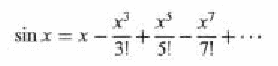singleton: A set with exactly one member.

singular matrix: A matrix for which an inverse matrix does not exist.

sinh: Shorthand for the hyperbolic function of hyperbolic sine.

sinusoidal: Of or relating to the sine curve.

SI units: International System of Units, a metric system of units of measurement.

size: 1. The number of edges in a graph, in the context of graph theory. (The number of vertices in a graph is the order of the graph.)

2. The number of units in a sample in sampling of a population in statistics.

skew: Also known as skewness, it is a measure of the asymmetry of a distribution.

skew curve: A space curve which is not entirely contained with a plane.

skew lines: Lines which are not coplanar. i.e. lines for which there is no plane which contains all of them.

skew-symmetric matrix: A matrix for which AT = -A.

slant height: Also known as slanted height, the length of a line segment between the apex and a (any) point on the circumference of the base of a cone.

slope: Also known as gradient, the ratio of the directed difference in y-coordinates to the directed difference in x-coordinates(calculated in the same order), between any 2 points on a line.

slope-intercept form: The expression of a line in equations in a form which clear shows the object's slope and (y-)intercept.

small circle: As opposed to a great circle. A circle of a sphere whose centre does not coincide with that of the sphere.

smooth: 1. A function which is infinitely differentiable.

2. A surface with no friction, i.e. a coefficient of friction of 0.

smooth curve: 1. The curve of a smooth function. Informally (and somewhat incorrectly), a curve representing a function which is (one-time) differentiable.

snowflake curve:  Also known as a Koch snowflake, it is a fractal formed by starting from an equilateral triangle, then replacing a line segment one third the length of and in the middle of each side, by two line segments of the same length pointing "outwards" (increasing area) so that the perimeter is a closed curve, the limiting case of the sequence formed by repeated these steps to each subsequent figure is known as the Koch snowflake.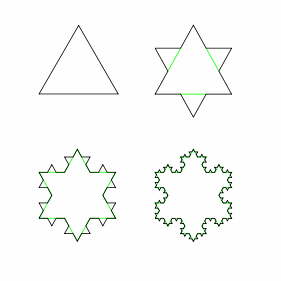solid: A 3-dimensional geometric object.

solid angle: A measure of the size of neighbourhood of a point (vertex) bounded by three or more lines (edges) coverging on the vertex in a polyhedron. It is the 3-dimensional analogue of a angle, defined to be the surface area of a polygon, defined by the intersection of the (extended) edges and the unit sphere.

The idea can then be extended to cover cases where the vertex of the solid figure is not connected to lines which can all be contained in a finite number of planes. (An irregularly shaped solid angle. e.g. the apex of a cone.)

solid geometry: Geometry which deals (mainly) with 3-dimensional geometric figures.

solid of revolution: A 3-dimensional geometric figure created by the interior of a surface of revolution.

solution of equations: The values of variables which satify the equations, i.e. all equations hold when substituted into the equations with no variables remaining.

solution set: The set of solutions a syste of equations or inequaties.

solve: To determine the solutions to a problem.

solve analytically: Finding the solution of a mathematical problem through algebraic manipulation.

solve graphically: Finding the solution of a mathematical problem through graphical manipulation.

space: A loosely defined idea of a set of elements with abstract algebraic structures which can be represented in, or identified with the physical concept of space.

space coordinates: Coordinates specifically used to identify the position of an (idealised) object in 3-dimensional space.

space curve: As opposed to a plane curve, a graph of 1-dimension in 3-dimensional space.

space-filling curve: The limit of a 1-dimensional curve which go through every point in a 2-dimensional geometric figure (usually a square).

spacetime: The 4-dimensional abstract space that models the 3 dimensions of space and 1 dimension of time in a way that interaction between these axes are more simply represented in such a model.

span: The set of vectors which can be expressed as linear combinations of a specified set of vectors in a vector space.

sparse matrix: A matrix consisting mostly of entries of zero.

Spearman's rank correlation coefficient: A measure of correlation between 2 variables by applying the Pearson product moment correlation coefficient on the ranks of the values, where equal values receive the mean of all (possible) ranks of the same value.

specific gravity:The relative density of a material to a specified reference material presented as a ratio..

speed: A scalar quantity that is the instantaneous rate of change of distance of an object (from a point on the tangent of the trajectory) with respect to time.

speed of light: SyThe speed that light travels at, in a vacuum, represented by the symbol c.

sphere: The locus of points in 3-dimensions which is of a particular specified distance from a specified point.

spherical angle: A solid angle formed by two arcs of great circles forming a closed curve. Equivalently, it is the solid angle formed by two planes intersecting each other.

spherical cone: A geometric solid marked by the area on a sphere within a circle (also on the sphere), and the lines joining the centre of the sphere to the circumference of the circle.

spherical coordinate system: A coordinate system where points are described by 3 numbers: the distance r of the point from the point of origin, the angle θ between the radial line and the z-axis and the angle φ of the projection of the radial line onto a plane perpendicular to the z-axis measured from the initial line (like that of polar coordinates) on the same plane. The coordinate system requires a reference initial line (x-axis), zenith axis (z-axis) and a reference sense of positive angle (conventionally anti-clockwise).

spherical polygon: A 2-dimensional (but not planar) geometric figure formed by 2 or more arcs of great circles forming a non-self-intersecting closed curve.

spheroid: An ellipsoid formed by stretching/compressing a sphere in one direction only.

spiral: A plane curve described by an equation in polar coordinates where the radius is a monotone function of the angle. Some common types of spirals are:

Archimedean spirals: r = kθ [Left diagram below]

hyperbolic spirals (reciprocal spirals): r = k/θ [Central diagram below]

parabolic spirals (Fermat's spirals): r = kθ1/2

logistic spirals (logarithmic spirals): r = pe [Right diagram below]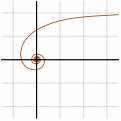spurious solution: Also known as an extraneous solution. Sometimes, used in the context of an unhelpful or unexpected solution, rather than an extra mathematical solution introduced due to incorrect manipulation of equations.

2. The number resulting from a number multiplying itself.

square free: An integer which does not contained repeated factors in its prime factorisation.

square matrix: A matrix where the number of rows and the number of columns are the same.

square number: A number which may be expressed as the square of an (non-negative or even positive, depending on the precise definition used) integer.

square root: The number whose square gives a specified number.

squaring the circle: The problem of creating a square, using compasses and straight edge, whose area is the same as that of a circle (also created using compasses and straight edge).

standard deviation: The square root of the mean of the squares of the distances of data points from the mean. It is thus the square root of the variance of a data set.

standard error: The standard deviation, or its estimate, of the sampling distribution of a statistic.

standard form: 1. The representation of a number through coefficients and exponents of a base number. e.g. scientific notations.

2. The representation of a mathematical object is a certain, conventionally agreed manner. e.g. a quadratic expression as ax2+bx+c and not (ax+b)x+c.

standardized random variable: A random variable transformed in the same way as a normally distributed random variable to a standard normal variable, for the purpose of comparison.

standard normal variable: A random variable which is normally distributed with a mean of 0 and a variance of 1 (i.e. standard normal distribution).

statics: The mathematical study of systems in equilibrium.

stationary point: Also known as a turning point or a critical point.

statistic: A function dependent only on the sample.

statistical significance: The probability that an observation/result occurs by chance.

statistics: The study of the various aspects of collection, organisation and analysis of data, as well as interpretation and presentation of results.

stem-and-leaf display: Also known as a stem-and-leaf diagram. A way of simplifying the representation of a quantiative data set by omitting repetitive elements and convey that information by position on the same stem (row).

For example,can be used to represent the data set of numbers: 7, 10, 11, 14, 19, 21, 27, 38, 41, 59

step discontinuity: Another class="d-title" name for a jump discontinuity.

Stirling's formula: Also known as Stirling's approximation, it is an approach to approximate the value of the factorial of an integer wherestochastic: Having the property of being random/non-deterministic.

stochastic model: A model which involves stochastic independent random variables.

stochastic process: A non-determnistic process, also known as a random process.

straight angle: The angle of 180°, also known as a flat angle.

straight line: Simply known in mathematics as a line. A one dimensional curve which is not straight is not considered a line

strain: The proportion/scale of deformation/transformation of an object in the direction of a force.

stratified sample: A sample where the proportions belonging to the various strata (cetegories) partitioning the population is manipulated so that the proportions are the same (or as close as possible) to that of the population..

stress: The amount of force per unit area within a cross section (perpendicular to the direction of force) of a body on which a force acts.

strict equivalence: Biconditional, implies and is implied by.

strict implication: The antecedent cannot be true with the consequent false at the same time.

strict inequality: An inequality which states the relationship between two unequal quantities (as being greater or less). i.e. a mathematical sentence with the symbols > or < between 2 expressions.

student's t-distribution: Also known simply as the t-distribution.

student's t-test: Also known simply as the t-test.

subclass: The class whose elements are also elements of a specified class.

subdiagonal: The set of elements directly below the leading diagonal of a matrix, i.e. mi,j where j + 1 = i.

subfactorial: Also known as the derangement number, it is the number of combinations where no elements appear in its (uniquely) assigned place, represented by !n, it is calculated by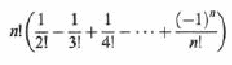subgraph: A graph which is a subset of another graph (in terms of https://itseducation.asia/mathematics/v.htm#vertex and edges), in the context of graph theory..

subjective probability: An interpretation to probability that the given (known) information in corresponds to belief of the observer.

subnormal: 1. A line segment which is defined by the normal (of a point to a curve).

2. The line segment or its length along the x-axis, between the intersection of the normal and the x-axis and the intersection of x-axis and a line through the conact point parallel to the y-axis. i.e. the projection of the normal between the x-intercept and the contact point, onto the x-axis.

subscript: Numbers and symbols written either smaller but with the bottom aligned or where both the top and bottom of the symbols below those of the baseline, in order to index object, indicate binomial coefficients etc.

sub-sequence: A sequence that is the subset of another specified sequence such that each term in the sub-sequence is identified by a unique term in the specified sequence (unique in the sense that no two terms in the subsequence are identified by the same term in the specified sequence), and an earlier/later term (relatively) within the subsequence is identified by an earlier/later term (relatively) within the specified sequence.

subset: A set which is completely contained within the specified set. i.e. a set such that any members of the subset is also a member of the specified set.

subspace: A vector space which is the subset of another specified vector space.

subtangent: 1. A line segment which is defined by the tangent (of a point to a curve).

2. The line segment or its length along the x-axis, between the intersection of the tangent and the x-axis and the intersection of x-axis and a line through the conact point parallel to the y-axis. i.e. the projection of the tangent between the x-intercept and the contact point, onto the x-axis.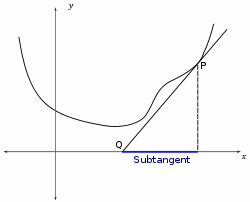subtend: To geometrically characterise (through indicating the limits etc.) by a geometric object.

subtraction: The inverse operation of addition. i.e. The process of finding the missing addend, given the sum (minuend) and another addend (subtrahend).

subtraction formulae: The trigonometric formulae relating the values of trigonometric functions with argument that is the difference of 2 angles, to the individual values of trigonometric functions of the 2 angles.

subtrahend: The known addend in the operation of subtraction, considered as inverse addition.

sum: The result from the operation of addition.

summand: 1. The terms of a summation.

2. Also known as an addend.

summation: The process of finding the sum of a sequence of quantities.

summation sign: The symbol Σ, an elarged Greek capital letter sigma to indicate the summation (of the terms decribed by the following expression substitued by values indicated above and below the summation sign) as in the following examplesup: A shorthand for supremum.

superdiagonal: The set of elements directly above the leading diagonal of a matrix, i.e. mi,j where i + 1 = j.

superscript: Numbers and symbols written either smaller but with the top aligned or where both the top and bottom of the symbols above those of the baseline, in order to index objects, indicate exponentiation,binomial coefficients, operation on sets etc.

superset: A set which completely contains the specified set. i.e. a set such that any members of the specified set is also a member of the superset.

supplemental chords: The chords which connect the endpoints of a diameter of a circle to a third point on the circumference.

supplementary angles: Angles whose sum is a straight angle (180°)000000.

supremum: Also known as the least upper bound.

surd: The representation of an irrational number by the use of radicals. e.g. √2 or 3 + √3.

surface: A geometric object which locally resembles (in the limiting case) a plane at almost all points.

surface of revolution: The surface traced by the rotation of a curve about a specified axis (of rotation).

surjection: A mapping whose codomain is precisely the image of the domain. i.e. for all elements in the codomain, there is an element of the domain which maps to it.

symmetric form: See line.

symmetric matrix: A matrix which is equal to its own transpose.

symmetric relation: A binary relation where xRy implies yRx for all x, y.

symmetry: self-similarity under transformation (of a part or the whole). e.g. reflections - reflective symmetry (mostly known simply as symmetry), rotations - rotational symmetry, enlargement - fractals

synthetic division: A method of dividing a polynomial by a linear expression analogous to the method of short division of numbers

system of equations: Also known as simultaneous equations.

A   B   C   D   E   F   G   H   I   J   K   L   M   N   O   P   Q   R   S   T   U   V   W   X   Y   Z

Genius is one percent inspiration and ninety-nine percent perspiration.